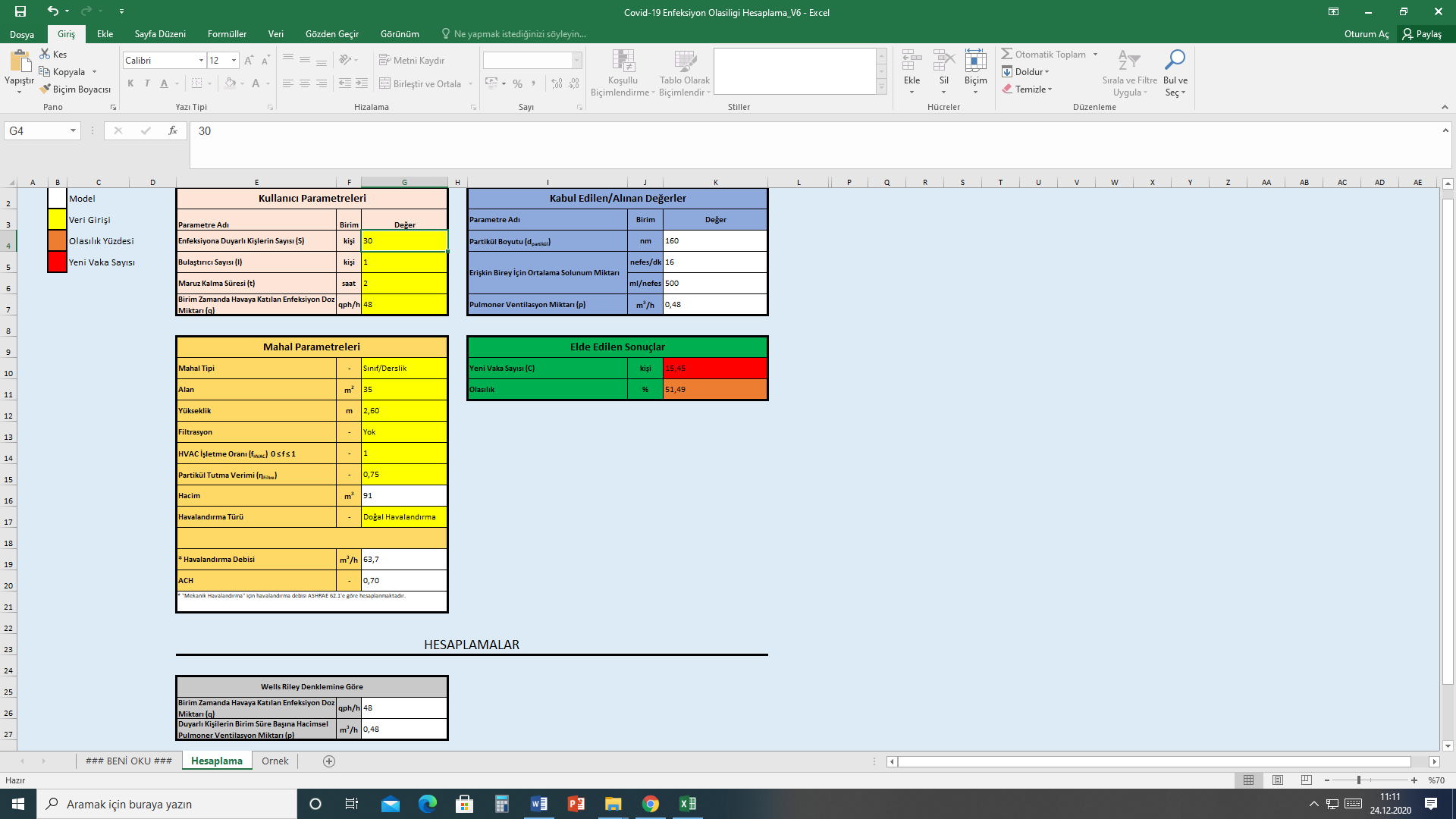• GAZİ ÜNİVERSİTESİ
News
Our University Has Developed a New Covid-19 Contamination Risk Calculating Method
1 April 2021 | 19:20

Our university has developed a method that can calculate the risk of contamination of Covid-19 and the number of new cases according to the indoor ventilation condition, the number of people, the number of infectious sources, and the length of its presence in the environment.

The risk of contamination was calculated as 47 percent if 30 people were together for 1 hour in a closed area of 35 square meters without ventilation and 1 person was contagious. Accordingly, 14 new cases were predicted at the end of this period.

In the case of natural ventilation, that is, if the windows in the space are open, under the same conditions the risk decreases to 30 percent and the number of new cases to 9. In the case of mechanical ventilation, under the same conditions the risk decreases to 2 percent while the number of new cases is estimated at 0.6.

Gazi University Faculty of Engineering Department of Mechanical Engineering faculty member Assoc. Prof. Dr. Zeki Yılmazoğlu stated that the calculation tool cannot give an exact infection risk result due to uncertainties, but it can make an estimate depending on the variables in the environment.

Assoc. Prof. Dr. Yılmazoğlu stated that in this calculating method, on the basis of the ventilation condition in the closed area, the number of people, the number of contaminating sources, the length of its presence in the environment and the amount of infection dose given per hour, "the amount of infection dose added to the air per unit time was left as user-defined and was evaluated as 48 quanta / hour in sample calculations”.  He stated however that in the literature it has been shown that for the new type of coronavirus (Covid-19) this value varies between 300 quanta / hour depending on the activity of people such as speaking loudly and singing. "

Yılmazoğlu expressed that they calculated the risk percentage by taking into account 3 different situations in the ventilation of closed areas, which they listed as "no ventilation", "mechanical ventilation" and "natural ventilation". Assoc. Prof. Dr. Yılmazoğlu said that based on these ventilation conditions and exposure time, the risk percentage and the number of new cases can be estimated by calculating the risk ratio. Yılmazoğlu pointed out that all variables made calculations taking into account the risk factors. He then gave the following information: “The probability percentage and the number of possible new cases were shown under the results obtained heading

The calculations were made by considering the respiratory characteristics in adults. The results will also be affected when there is a super spreader in the environment, since the amount of dose delivered to the environment per unit time will change. This calculating tool reveals an approach with the condition of mask wearing, practising physical distance and good hygiene and shows the importance of ventilation in the prevention of the epidemic.

The risk of contamination was calculated as 47 percent if 30 people were together for 1 hour in a closed area of 35 square meters with no ventilation, a height of 2.6 meters and 1 person was contagious. At the end of this period, 14 new cases were predicted. With natural ventilation, that is, if the windows in the space were open, under the same conditions the risk was calculated as 30, and it was estimated that 9 new cases could occur after 1 hour. In the case of mechanical ventilation, under the same conditions the risk fell to 2 percent, and the number of new cases was calculated to be 0.6.

Yılmazoğlu stated that the calculation tool can be used to predict the percentage of infection risk and the number of new cases in a closed area according to Wells-Riley models. Emphasizing that many factors, such as the limitations of the calculation tool, the presence of a super spreader in the environment, the condition of sensitive people, need to be taken into consideration while using the technique, Yılmazoğlu said, "The study once again revealed the importance of ventilation in preventing contamination in closed areas. While the risk is around 30 percent only in the case of a leakage (windows closed) in 40 minutes, this risk is reduced to 1 percent with mechanical ventilation"

Pointing out that different spaces and the ventilation flow rates required for these spaces can be selected in the calculation tool, Yılmazoğlu also stated that under a mechanical ventilation situation, associating the filtration retention efficiency with the risk percentage also provides a prediction for filter selection.